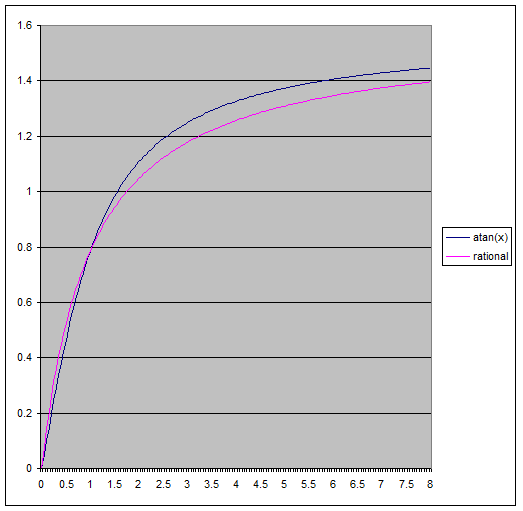## Faster Fog

Cedrick at Lucas suggested some nice optimisations for the in-scattering equation I posted last time.

$L_{s} = \frac{\sigma_{s}I}{v}( \tan^{-1}\left(\frac{d+b}{v}\right) - \tan^{-1}\left(\frac{b}{v}\right) )$

But we can remove one of the two inverse trigonometric functions by using the following identity:

$\tan^{-1}x - \tan^{-1}y = \tan^{-1}\frac{x-y}{1+xy}$

Which simplifies the expression for $L_{s}$ to:

$L_{s} = \frac{\sigma_{s}I}{v}( \tan^{-1}\frac{x-y}{1+xy} )$

With $x$ and $y$ being replaced by:

$\begin{array}{lcl} x = \frac{d+b}{v} \\ y = \frac{b}{v}\end{array}$

So the updated GLSL snippet looks like:

float InScatter(vec3 start, vec3 dir, vec3 lightPos, float d)
{
vec3 q = start - lightPos;

// calculate coefficients
float b = dot(dir, q);
float c = dot(q, q);
float s = 1.0f / sqrt(c - b*b);

// after a little algebraic re-arrangement
float x = d*s;
float y = b*s;
float l = s * atan( (x) / (1.0+(x+y)*y));

return l;
}


Of course it's always good to verify your 'optimisations', ideally I would take GPU timings but next best is to run it through NVShaderPerf and check the cycle counts:

Original (2x atan()):

Fragment Performance Setup: Driver 174.74, GPU G80, Flags 0x1000
Results 76 cycles, 10 r regs, 2,488,320,064 pixels/s


Updated (1x atan())

Fragment Performance Setup: Driver 174.74, GPU G80, Flags 0x1000
Results 55 cycles, 8 r regs, 3,251,200,103 pixels/s


A tasty 25% reduction in cycle count!

Another idea is to use an approximation of atan(), Robin Green has some great articles about faster math functions where he discusses how you can range reduce to 0-1 and approximate using minimax polynomials.

My first attempt was much simpler, looking at it's graph we can see that atan() is almost linear near 0 and asymptotically approaches pi/2.Perhaps the simplest approximation we could try would be something like:

$\tan^{-1}(x) \approx min(x, \frac{\pi}{2})$

Which looks like:float atanLinear(float x)
{
return clamp(x, -0.5*kPi, 0.5*kPi);
}

// Fragment Performance Setup: Driver 174.74, GPU G80, Flags 0x1000
// Results 34 cycles, 8 r regs, 4,991,999,816 pixels/s


Pretty ugly, but even though the maximum error here is huge (~0.43 relative), visually the difference is surprisingly small.

Still I thought I'd try for something more accurate, I used a 3rd degree minimax polynomial to approximate the range 0-1 which gave something practically identical to atan() for my purposes (~0.0052 max relative error):float MiniMax3(float x)
{
return ((-0.130234*x - 0.0954105)*x + 1.00712)*x - 0.00001203333;
}

float atanMiniMax3(float x)
{
// range reduction
if (x < 1)
return MiniMax3(x);
else
return kPi*0.5 - MiniMax3(1.0/x);
}

// Fragment Performance Setup: Driver 174.74, GPU G80, Flags 0x1000
// Results 40 cycles, 8 r regs, 4,239,359,951 pixels/s


Disclaimer: This isn't designed as a general replacement for atan(), for a start it doesn't handle values of x < 0 and it hasn't had anywhere near the love put into other approximations you can find online (optimising for floating point representations for example).

As a bonus I found that putting the polynomial evaluation into Horner form shaved 4 cycles from the shader.

Cedrick also had an idea to use something a little different:

$\tan^{-1}(x) \approx \frac{\pi}{2}\left(\frac{kx}{1+kx}\right)$

This might look familiar to some as the basic Reinhard tone mapping curve!  We eyeballed values for k until we had one that looked close (you can tell I'm being very rigorous here), in the end k=1 was close enough and is one cycle faster :)float atanRational(float x)
{
return kPi*0.5*x / (1.0+x);
}

// Fragment Performance Setup: Driver 174.74, GPU G80, Flags 0x1000
// Results 34 cycles, 8 r regs, 4,869,120,025 pixels/s


To get it down to 34 cycles we had to expand out the expression for x and perform some more grouping of terms which shaved another cycle and a register off it.  I was surprised to see the rational approximation be so close in terms of performance to the linear one, I guess the scheduler is doing a good job at hiding some work there.

In the end all three approximations gave pretty good visual results:

Original (cycle count 76):MiniMax3, Error 8x (cycle count 40):

Rational, Error 8x (cycle count 34):

Linear, Error 8x (cycle count 34):